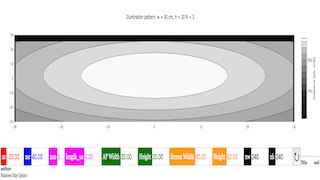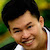Intro Page

Developed by E. Behringer

This set of exercises guides the student in exploring computationally the behavior of light patterns and shadows generated by simple light sources together with apertures in thin, opaque barriers. It requires the student to generate, and describe the results of simulating, light patterns and shadows. Diffraction is ignored. The numerical approach used is summing over a two-dimensional spatial grid while applying a logical mask (‘transparency function’). Please note that this set of computational exercises can be affordably coupled to simple experiments with small light bulbs and apertures cut into (or barriers cut out of) opaque paper sheets. A possible extension is to compare the predicted light patterns to experimental measurements. This set of exercises could be incorporated as an initial activity in an intermediate optics laboratory.

 Subject Area Waves & Optics First Year and Beyond the First Year Python Students who complete this set of exercises will be able to predict and visually represent the irradiance distribution at a screen generated by a point light source and an aperture (Exercise 1); predict and visually represent the irradiance distribution at a screen generated by multiple point light sources and an aperture (Exercise 2); predict and visually represent the irradiance distribution at a screen generated by multiple point light sources and a complex aperture (Exercise 3); predict and visually represent the irradiance distribution at a screen generated by a two-dimensional array of point light sources and an aperture (Exercise 4);, and predict and visually represent the irradiance distribution at a screen generated by a two-dimensional array of point light sources and an opaque barrier (Exercise 5). 120 min

#

#

# A linear array of N point sources located a specified distance from

# a rectangular aperture that is centered on the origin.

# A screen is located a specified distance from

# the aperture.

#

# This file will generate a filled contour plot of

# the irradiance of light reaching the screen

# versus lateral coordinates (x,y)

#

# Written by:

#

# Ernest R. Behringer

# Department of Physics and Astronomy

# Eastern Michigan University

# Ypsilanti, MI 48197

# (734) 487-8799

# This email address is being protected from spambots. You need JavaScript enabled to view it.

#

# 20160112-13 original code by ERB

# 20160609 clean up by ERB

#

# import the commands needed to make the plot

from pylab import xlabel,ylabel,xlim,ylim,axis,show,contourf,colorbar,figure,title

from matplotlib import cm

# import the command needed to make a 1D array

from numpy import meshgrid,absolute,where,zeros,linspace

# inputs

zs = -20.0 # distance between the source and aperture [cm]

zsc = 40.0 # distance between the screen and aperture [cm]

nso = 3 # number of point sources

length_so = 4.0 # length of the array of point sources [cm]

ap_width = 4.0 # aperture width [cm]

ap_height = 3.0 # aperture height [cm]

screen_width = 60.0 # screen width [cm]

screen_height = 60.0 # wcreen height [cm]

nw = 240 # Number of screen width intervals

nh = 240 # Number of screen height intervals

# initialize needed arrays to zero

rso = zeros((nso,2)) # (x,y) source positions [cm,cm]

screen_x = zeros((nw+1,nh+1)) # x-coordinates of screen points

screen_y = zeros((nw+1,nh+1)) # y-coordinates of screen points

rsq = zeros((nw+1,nh+1)) # r squared values

x0 = zeros((nw+1,nh+1)) # x-coordinates of intersections at aperture plane

y0 = zeros((nw+1,nh+1)) # y-coordinates of intersections at aperture plane

# create 1D arrays to create a meshgrid for contour plotting

screen_xx = linspace(-0.5*screen_width,0.5*screen_width,nw+1)

screen_yy = linspace(-0.5*screen_height,0.5*screen_height,nh+1)

# generate the meshgrid of screen points

screen_xx, screen_yy = meshgrid(screen_xx,screen_yy)

# Set up point source coordinates

# Here, the sources are arranged

for i in range (0,nso):

for j in range (0,2):

if j==0:

rso[i,j] = -0.5*length_so + i*length_so/(nso-1)

else: # j = 1 and the y-coordinate is

rso[i,j] = 0.0

# Calculate grid increments

deltaw = screen_width/nw # grid increment, width [cm]

deltah = screen_height/nh # grid increment, height [cm]

# Define the array of screen x and screen y values

for i in range (0,nw+1):

for j in range (0,nh+1):

screen_x[i,j] = -0.5*screen_width + deltaw*i

screen_y[i,j] = -0.5*screen_height + deltah*j

# Calculate the irradiance at each screen point

for k in range (0,nso):

for i in range (0,nw+1):

for j in range (0,nh+1):

# First calculate square of distance from source to screen

rsq = (screen_x[i,j]-rso[k,0])**2 + (screen_y[i,j]-rso[k,1])**2 + (zsc - zs)**2

# Calculate x and y coordinates at the aperture

x0[i,j] = rso[k,0] + abs(zs)*(screen_x[i,j] - rso[k,0])/(zsc - zs)

y0[i,j] = rso[k,1] + abs(zs)*(screen_y[i,j] - rso[k,1])/(zsc - zs)

# Check if the coordinates fall within the aperture

# make a filled contour plot of the period vs overlap and length ratios

figure()

title('Illumination pattern: $$w =$$%s cm, $$h =$$%s; $$N =$$%d'%(ap_width,ap_height,nso))

axis('equal')

xlim(-0.5*screen_width,0.5*screen_width)

ylim(-0.5*screen_height,0.5*screen_height)

xlabel("$$x$$ [cm]")

ylabel("$$y$$ [cm]")

show()

Exercise 2

### EXERCISE 2: IRRADIANCE DUE TON$N$POINT SOURCES WITH A RECTANGULAR APERTURE

If instead of one point source, suppose $N$ point sources are uniformly spaced and also arranged along a line segment of total length $L$ that is parallel to the $x$-axis, as shown below.

Calculate the irradiance at the screen for the aperture of Exercise 1 if one source is on the symmetry axis, $L=4.0$ cm, and: (a) $N=3$; (b) $N=11$; (c) and $N=101$. How many distinct shadow regions (areas characterized by different irradiance) appear in each case?

### Translations

Code Language Translator Run### Software Requirements

SoftwareRequirements

 Android iOS Windows MacOS with best with Chrome Chrome Chrome Chrome support full-screen? Yes. Chrome/Opera No. Firefox/ Samsung Internet Not yet Yes Yes cannot work on some mobile browser that don't understand JavaScript such as..... cannot work on Internet Explorer 9 and below

### CreditsFremont Teng; Loo Kang Wee; based on codes by E. Behringer

Version: